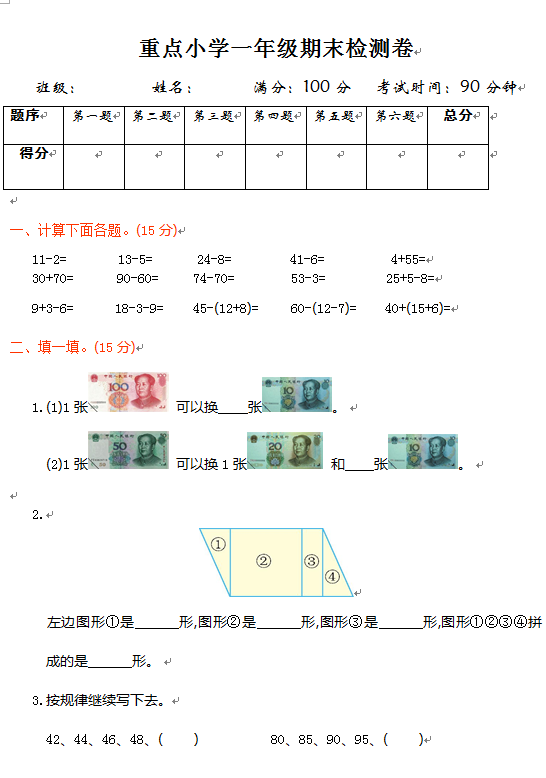11-2=　　　 13-5=　　　24-8=　　　　41-6=　　　　 4+55=

30+70=        90-60=       74-70=           53-3=          25+5-8=

9+3-6=       18-3-9=      45-(12+8)=     60-(12-7)=　  40+(15+6)=

【DOC文档3页】重点小学一年级下册-数学期末检测卷（1）含答案 A4电子版资料_可直接打印_会员免费下载评论信息